# How to calculate textbox

This blocks can convert alphabet to number?

and

How can i calculate this …

I don’t know how to calculate text

Use the block `length of the` block

How can i use it?

I want to calculate this
ex) HE+G/N=?

only text or all the things including + / =?

no…

all of four rule calculations

including -

If i type the same thing(HE+G/N=?) in the text box and clcik button, it will return total length as 8 in the label 1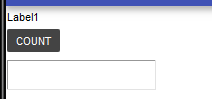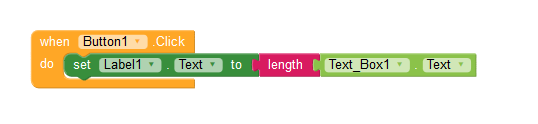in this example

H = 3 , E = 6 , G = 8 , N = 4

result = 36+8/4

finally i got 38

then you have to use maths block… wit i will show you

Working fine…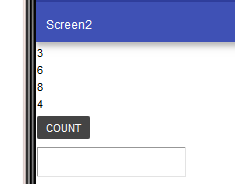Umm…

I’m sorry to my mistaken explanation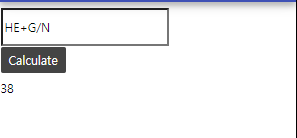I want input “HE+G/N” after i click Calculate button then return 38

I don’t know my blocks is right and How to calculate in textbox

Instead why dont you use like this…

I dont think your idea will work…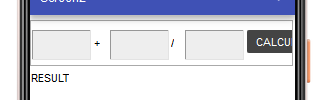Because four rule calculations aren’t fix

There is EAR-7T-H and EA-R+TH and E4-A+R*2-TH Etc…

Don’t know what you are expecting…

Anyway, set up four procedure for the above mentioned four rules based on my first explanatory.

On clicking the button, you decide which rule need to apply and get result according to that.

I’m so sorry about my short English

I don’t know what the rule to use

1. input the textbox like this example “GAR-DEN”
2. click ‘calculate’ button
3. Convert “GAR-DEN” to “409-268”
4. Calculate this expression
5. output “152” to result label

In this process i don’t know my convert block is right and how can calculate converted text

Thanks to explain but i still don’t understand
Sorry (T_T)

I hope you have the value in the label of G, A ,R D, E and N. If so, If you use the block JOIN as mentioned in this post you will get GAR = 409 and work accordingly…

I’m sorry but the positions of the four rule calculations are all different location. How do I calculate them?

Ok show what you have tried? Then myself or other will help you .

This topic was automatically closed 30 days after the last reply. New replies are no longer allowed.